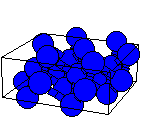Crystal Lattice Structures: Creation Date: 26 Aug 1998 Last Modified: 13 Dec 2005

# The γSe (A8) Crystal StructureYou can now

• Donohue refers to this as the α Se structure, calling α Se and β Se ``monoclinic α'' and ``monoclinic β,'' respectively.
• When x = 1/3 this reduces to the Ai (β Po) or A10 (α Hg) structure.
• If, in addition, c = 61/2 a, then the structure becomes fcc (A1).
• On the other hand, if c = (3/2)1/2 a, then the structure becomes simple cubic (A_h).

• Prototype: γSe
• Pearson Symbol: hP3
• Strukturbericht Designation: A8
• Space Group: P3121 (Cartesian and lattice coordinate listings available)
• Number: 152
• Reference: Villars and Calvert, Pearson's Handbook, vol. IV, 5215.
• Other Systems with this Structure: Te, SeTe, Se3Te
• Primitive Vectors:  A1 = ½ a X - ½ 31/2 a Y A2 = ½ a X + ½ 31/2 a Y A3 = c Z
• Basis Vectors:  B1 = x A1 + 1/3 A3 = ½ a x X - ½ 31/2 a x Y + 1/3 c Z (Se) (3a) B2 = x A2 + 2/3 A3 = ½ a x X + ½ 31/2 a x Y + 2/3 c Z (Se) (3a) B3 = -x A1 - x A2 = - a x X (Se) (3a)

### See these vectors in LaTeX output format.

Go back to the face-centered cubic and related structures page.

Go back to Crystal Lattice Structure page.

 Structures indexed by: This is a mirror of an old page created at theNaval Research LaboratoryCenter for Computational Materials ScienceThe maintained successor is hosted at http://www.aflowlib.org/CrystalDatabase/ and published as M. Mehl et al., Comput. Mater. Sci. 136 (Supp.), S1-S828 (2017).